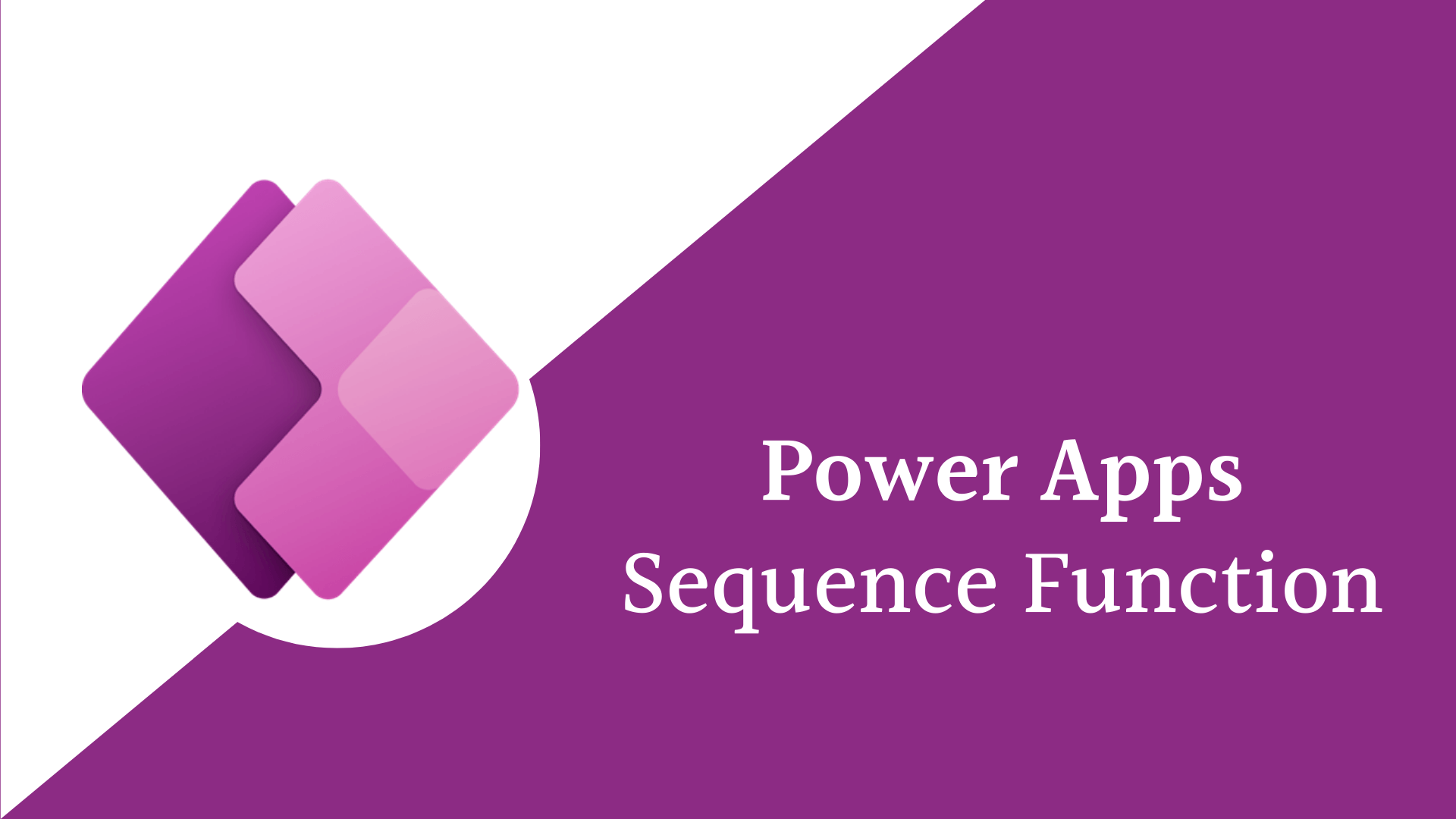PowerApps Sequence Function

## How to use Sequence function in PowerApps?

• Post author:
• Post category:Power Apps

In this blog post, we will discuss what is the Sequence function in PowerApps, and how to use Sequence function in PowerApps with examples?

## What is Sequence function in PowerApps?

• The PowerApps Sequence function generates a single column table of sequential numbers, such as 1, 2, 3,4,etc.
• Sequence is limited to 50,000 records.
• The name of the column that returned by Sequence function is Value.

## Syntax

SequenceRecords [, Start [, Step ] ] )

• Records – Is Required value and it is the number of records to create. Must be in the range 0 to 50,000.
• Start – Is Optional and it is represent the starting number for the sequence. Default is 1.
• Step – Is Optional it represent the increment for each successive number in the sequence. Step can be negative to count down from the Start. Default is 1.

Example1: Sequence(6) The Output will be [1,2,3,4,5,6]

This is how PowerApps generate sequence number using the Sequence function .

Example2: generate Sequence number and count down from 9 and incrementing by -1, In this case the output will be a table of 10 records starting from 9 . output [9,8,7,6,5,4,3,2,1,0]

``Sequence(10,9,-1)``

Example3: Generate 4 column table starting with 24 and incremented by 2 the output will be [24,26,28,30]

``Sequence( 4,24,2)``

Example4: It can be used with ForAll function, the ForAll function can also be used to transform the value into other data types and return a new table

The following formula returns a table of the next 5 days

``````//you can use this formula
ForAll( Sequence( 5 ), DateAdd( Today(), Value, Days ) )
//or you can use this both are the same
ForAll(Sequence(5), Today() + Value)
``````

Example5: In this example we will use ForAll function with Sequence function to generate a gallery of blank textboxes that specified by the user in the TextInput2 textbox. It will generate the gallery according to the user inputs.

``ForAll(Sequence(Value(TextInput2.Text)), Blank())``

Example6: You can Generate random Numbers , the following example generates a 4 record table of random numbers.

``ForAll( Sequence( 4 ), Rand() )``